# Colour Morphology

#### Introduction

Although mathematical morphology can be readily applied to multi-variate images, we find that morphological filters produce pixel values in the output image that are not present in the input image. For example, morphologically filtering a colour image will introduce new colours not contained in the input image. It is impossible to do quite simple filtering under these conditions. We have shown that by artificially imposing an invertible ordering of the partially ordered multi-variate space, it is possible to morphological filter a multi-variate image while introducing no new pixel values .

#### Mathematical Morphology and Lattice Theory

One of the basic ideas in mathematical morphology is that the range of all possible images constitutes a complete lattice. We can demonstrate the notion of a complete lattice using the simple example of a small 3 by 1 binary image with pixel values 0 and 1 . In all, there exist eight possible 3 by 1 binary images, as shown in Fig. 1 below.##### Figure 1: Example of a complete lattice: the set of all 3 by 1 binary images.

These images can be ordered using a partial ordering relation < , as indicated by the lines in this figure. For example, 1 0 0 < 1 1 0. We can say this because each pixel in the image 1 0 0 is less than or equal to its corresponding pixel in the image 1 1 0 . The ordering is only partial because some pairs of images cannot be ordered, for example 0 1 0 and 1 0 1 . Here we have neither 0 1 0 < 1 0 1 nor 1 0 1 > 0 1 0 and so no line has been drawn between these two images. The concept of an infimum and a supremum stem from the partial ordering relation. The infimum of a set of images is defined as the greatest lower bound of the images, which for binary images is simply the pixel-wise minimum of the images. For example, the infimum of 1 0 0 and 1 1 0 is 1 0 0 and the infimum of 1 1 0 and 0 1 1 is 0 1 0 . In a similar way, we define the supremum of a set of images as the least upper bound, or the pixel-wise maximum of the images. If the supremum and infimum exist for any collection of images taken from the lattice of all images, then that lattice is called a complete lattice. The set of 3 by 1 binary images is such a lattice. More generally, the set of all binary images is a complete lattice, as is the set of all grey-scale images.

In mathematical morphology, we consider an image filter as a mapping performed within a complete lattice; the filter maps one lattice element (the input image) to another lattice element (the output image). The key insight provided by mathematical morphology is to construct these image filters from what are called erosions and dilations . Erosions and dilations have proved to be very powerful and can be combined to yield effective image filters with desirable filtering properties. For example, idempotence is generally regarded as a useful property for filters that are used to remove noise, because it guarantees that a second pass of the filter has no further effect. An idempotent image filter can be constructed by either cascading an erosion with its dual dilation (an opening ) or cascading a dilation with its dual erosion (a closing ).

An erosion translates the image around through the points in a window B and takes the point-wise infimum of all the translated images. The result at any given point in the output image is therefore given by an infimum of the vectors in the window, which is just the component-wise minimum of these vectors. As shown below in Fig. 2 for a window of size 5 by 5, the resultant vector is a mixture of the various vectors in the window and will not usually correspond to any particular one of these vectors. Rather, the mixture will be a new vector not contained in the window.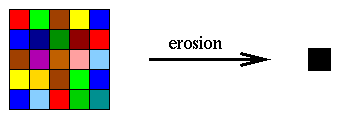##### Figure 2: An erosion produces a new colour not contained in the window.

Similarly, a dilation at any given point is given by the supremum of the vectors in the window B , which is just the component-wise minimum of these vectors.

#### Filtering and the Generation of New Colour

We can show with an example that under these conditions it is impossible to do quite simple morphological filtering. Consider trying to remove the small red balls from a green background. The desired result is shown in Fig. 3b.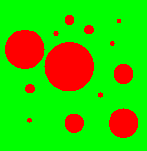(a) (b)
##### Figure 3: Trying to remove small red balls on a green background.

Any series of erosions and dilations we apply to the colour image can be applied to the components separately, due to the fact that these filters commute with infimum and supremum respectively. The separate red and green components are shown in Figs. 4a and 4b respectively. The aim is to remove the small balls in the red component while filling in the small balls in the green component. We can try a cascade of an erosion followed by a dilation (an opening). Applying the same erosion to each component we get the result in Figs. 4c and 4d for the green and red respectively. Following with the dilation we get the final result in Figs. 4e and 4f. The small balls in the green component have been removed but not in the red component.(a) (b) (c)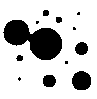(d) (e) (f)
##### Figure 4: Processing the separate components of the image in Fig. 3a.

Combining these two components we find that, instead of being removed, the small red balls have been replaced by small black balls, as shown in Fig. 5.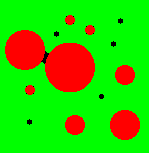##### Figure 5: A new colour is produced by the filtering.

A closing would give a similar result, only small red balls would be replaced by small white balls. Another less orthodox approach would be to apply different filters to different components rather than the same filter to all components. However we can show that by introducing blue balls to the scenario this will not work either.

In essence, the reason that the morphological approach fails is that the colours red and green are vectors which cannot be ordered and so the supremum or infimum of the two is a mixture of both the colours. However, our approach is to impose an artificial ordering that, for example, the colour green is greater than the colour red. In such case, the supremum of red and green would be green and not a mixture of the two colours. Similarly, the infimum would be red and not a mixture of the two colours. This approach would allow the small red balls in Fig. 3a to be removed because they could be replaced by the colour green and not a mixture of red and green.

#### Morphological Filters with No New Colour

The partial ordering `<‘ that exists between multi-variate pixels is defined by the following conditions , where P1, P2 and P3 are any combination of multi-variate pixel values: (i) P1 < P1 (reflective law); (ii) P1 < P2 and P2 < P1 implies that P1=”P2″ (anti-symmetric law); (iii) P1 < P2 and P2 < P3 implies that P1 < P3 (transitive law). However, to impose a total ordering, we need to define a new total ordering relation `<<‘ with the above three properties plus the additional property: (iv) P1 << P2 or P2 << P1. This artificially imposes a total ordering of any two partially ordered lattice pixels and, when combined with conditions (i)-(iii), totally orders the lattice of multi-variate pixels.

There are many ways to total order a multi-variate pixel lattice according to conditions(i)-(iv). A simple example is the so-called lexicographic order: when comparing two pixels P1 and P2, begin by comparing their first components; the vector with the biggest first component is chosen. If the first components are equal, select the vector with the biggest second component, and so on. In this way, any two pixels P1 and P2 can be ordered so that P1 << P2 or P2 << P1, thus satisfying condition (iv). Perhaps a more interesting example is for colour images, where we can base the ordering on the luminosity, hue and saturation scheme. In this case, the pixel with the greatest luminosity (red + green + blue value) is chosen. If luminosity values are equal, then the pixel with the greatest saturation (minimum of red, green and blue values) is chosen. If these values are equal then we turn to the hue. In general however, we would anticipate that the particular image and noise to be filtered should determine the type of ordering used.

It is not difficult to see that using erosion and dilation in conjunction with a total ordering `<<‘ will generate no new colours. For example, the result of an erosion at any given point in the output image is given by an infimum of various translated colours. Under the ordering relation `<<‘, these colours are totally ordered so that infimum will be one of the actual colours (and not a mix of all the colours). Therefore, the only colours in the output image will be those obtained from translations of the input colours; no new colours will be generated. A similar result holds for dilation, and combinations of erosion and dilation such as openings and closings.

We can apply a total ordering to the problem of removing small red balls on a green background discussed above, where the process is shown in Fig. 6. To remove the balls 6a, we can use any total ordering of the colour pixel space, as long as the colour red is ordered lower than the colour green. We then apply a dilation directly on the colour image using a window B slightly bigger than the size of the small balls. The result is shown in Fig. 6b. If we then follow this with an erosion using the same structuring element we get the result shown in Fig. 6c; the small red balls have been removed without generating new colours. It is interesting to note that there is actually a dual solution to the problem by ordering the colour red higher than the colour green, instead of lower. In this case, an erosion followed by a dilation will remove the small red balls.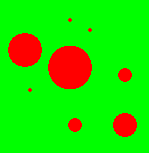(a) (b) (c)

#### Orderings for Grey-Scale Images

The concept of imposing an artificial ordering may also have application to grey-scale images, where the ordering is already a total ordering. For example, although the `natural’ ordering 0 <1 < 2 < … < 255 is always used for 8-bit images, we can also try artificial total orderings (of which there are 512! possibilities). We can consider for example the filtering of impulse noise from an image, where the `salt’ is of value 255 and the `pepper’ of value 0. Albeit somewhat contrived, this example illustrates how alternative total orderings may be of use in grey-scale image filtering. In 7a is the original image and in Fig. 7b is the image with noise added. To filter this noise we can apply a 3 x 3 median filter, as shown in in 7c. Although this operation is quite effective, it does not have the desirable property of idempotence that morphological filters have. In 7d we illustrate a typical idempotent morphological approach; we first filter the pepper using a small 3 x 3 closing and then the salt using a 3 x 3 opening. The initial closing has joined together some of the `salt’ noise and this is not removed by the subsequent opening. This is a classic problem in morphology when the noise to be filtered is both bright and dark relative to the background. An opening followed by a closing gives a similarly unsatisfactory result. In 7e is a result produced by re-defining the ordering of the pixel lattice, where we use the ordering 0 < 255 < 1 < 2 < … < 254 instead of the natural ordering. Applying a single 3 x 3 closing in conjunction with this ordering removes the salt and the pepper noise simultaneously, because the salt is considered to be as dark as the pepper. This operation gives a similar result to the median filter but is idempotent.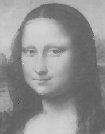(a) (b) (c)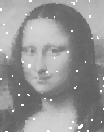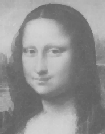(d) (e)

#### References

 Jones R and Talbot T, `Morphological filtering for colour images with no new colours’, in IVCNZ’96 (Image and Vision Computing New Zealand), pp. 149-154, Lower Hutt.  Serra J, `Image analysis and mathematical morphology. Volume 2: Theoretical advances’, Academic Press, London, 1988